# KSEEB SSLC Class 10 Maths Solutions Chapter 2 Triangles Ex 2.5

KSEEB SSLC Class 10 Maths Solutions Chapter 2 Triangles Ex 2.5 are part of KSEEB SSLC Class 10 Maths Solutions. Here we have given Karnataka SSLC Class 10 Maths Solutions Chapter 2 Triangles Exercise 2.5.

## Karnataka SSLC Class 10 Maths Solutions Chapter 2 Triangles Exercise 2.5

Exercise 2.5 Class 10 Maths Triangles Question 1.
Sides of triangles are given below. Determine which of them are right triangles. In case of a right triangle, write the length of its hypotenuse.
i) 7 cm, 24 cm, 25 cm,
ii) 3 cm, 8 cm, 6 cm.
iii) 50 cm, 80 cm, 100 cm.
iv) 13 cm, 12 cm, 5 cm.
Solution:
In ⊥∆ABC, ∠B = 90°.
Let AB = a, BC = b, Hypotenuse AC = c then
AC2 = AB2 + BC2
c2 = a2 + b2
∴ Here diagonal is the greatest side.
i) a, b,c
7 cm, 24 cm, 25 cm,
c2 = a2 + b2
252 = (7)2 + (24)2
625 = 49 + 576
625 = 625
625 = 49 + 576 625 = 625
∴ This is right angled triangle.
Measurement of Hypotenuse, c = 25 cm.

ii) a c b
3 cm, 8 cm, 6 cm.
c2 = a2 + b2
82 = (3)2 + (6)2
64 = 9 + 36
64 ≠ 45
∴ These are not sides of right angled triangle.

iii) a b c
50 cm, 80 cm, 100 cm.
c2 = a2 + b2
1002= (50)2 + (80)2
10000 = 2500 + 6400
10000 ≠ 8900
∴ These are not sides of right angled triangle.

iv) a b c
12 cm, 5 cm, 13 cm,
c2 = a2 + b2
132 = (12)2 + (5)2
169 = 144 + 25
169 = 169
∴ These are sides of right angled triangle.
Measurement of Hypotenuse =13 cm.

Triangles Exercise 2.5 Question 2.
PQR is a triangle right angled at P and M is a point on QR such that PM ⊥ QR. Show that PM2 = QM.MR.
Solution: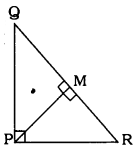Data: PQR is a triangle 9 right angled at P and M is a point on QR such that PM ⊥ QR.
To Prove: PM2 = QM.MR
Proof: In ⊥D QPR, ∠P = 90°,
QR2 = QP2 + PR2 ……………. (i)
In ⊥D PMQ, ∠M = 90°
QP2 = PM2 + QM2 ………. (ii)
In ⊥D PMR, ∠M = 90°
PR2 = PM2 + MR2 ……………. (iii)
By Adding eqn. (ii) and (iii)
QP2 + PR2 = PM2 + QM2 + PM2 + MR2
QR2 = 2PM2 + QM2 + MR2 (. Eqn. (i))
(QM + MR)2 = 2PM2 + QM2 + MR2
QM2 + MR2 + 2QM.MR = 2PM2 + QM2 + MR2
QM2 – QM2 + MR2 – MR2 + 2QM.MR = 2PM2
2QM.MR = 2PM2
2PM2 = 2QM.MR
∴ PM2 = QM.MR

Triangles Class 10 Exercise 2.5 Question 3.
In the following figure, ABD is a triangle right angled at A and AC ⊥BD. Show that
i) AB2= BC.BD
ii) AC2 = BC.DC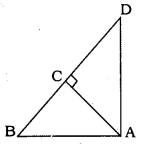Solution:
Data: In ∆ABD, ∠A = 90°,
AC ⊥ BD.
To Proved: AB2 = BC.BD
ii) AC2 = BC.DC

i) AB2 = BC.BD
$$\frac{\mathrm{AB}}{\mathrm{BD}}=\frac{\mathrm{BC}}{\mathrm{AB}}$$
∴ AB2= BC × BD.

ii) AC2 = BC.DC
∆BCA ~ ∆ACD
$$\frac{\mathrm{AB}}{\mathrm{AD}}=\frac{\mathrm{AC}}{\mathrm{CD}}=\frac{\mathrm{BC}}{\mathrm{AC}}$$
∴ AC × AC = BC × CD
∴ AC2 = BC × CD

$$\frac{\mathrm{AD}}{\mathrm{BD}}=\frac{\mathrm{CD}}{\mathrm{AD}}=\frac{\mathrm{AC}}{\mathrm{AB}}$$
∴ AD2 = BD × DC

Triangles Class 10 Exercise 2.5 Solutions Question 4.
ABC is an isosceles triangle right angled at C. Prove that AB2 = 2AC2.
Solution:
Data: ABC is an isosceles triangle right angled at C.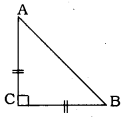To Prove: AB2 = 2AC2.
In ⊥∆ACB, ∠C = 90°
∴ AB2= AC2 + BC2
(∵ Pythagoras Theorem)
AB2 = AC2 + AC2 (∵ AC = BC)
AB2 = 2 AC2.

10th Maths Triangles Exercise 2.5 Question 5.
ABC is an isosceles triangle with AC = BC. If AB2 = 2AC2, prove that ABC is a right-angled triangle.
Solution:
Data: ABC is an isosceles triangle with AC = BC.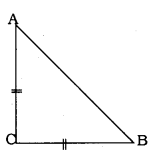AB2 = 2 AC2.
To Prove: ∆ABC is a right angled triangle
AB2 = 2AC2 (Data)
AB2 = AC2 + AC2
AB2 = AC2 + BC2 (∵ AC = BC)
Now, in ∆ABC, square of one side is equal to squares of other two sides.
∆ABC is a right angled triangle, Opposite angle to AB, i.e., ∠C is 90°.

KSEEB Solutions For Class 10 Maths Triangles Question 6.
ABC is an equilateral triangle of side 2a. Find each of its altitudes.
Solution:
Data: ABC is an equilateral triangle of side 2a.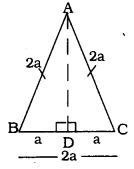To Prove: Altitude of ∆ABC,
In equilateral triangle
D bisect base.
∴ AB = BC = CA = 2a. 3 D a
If BC = 2a,
$$\frac{1}{2} \mathrm{BC}=\mathrm{a}$$ unit
∴ BD = DC = a.
Now, in ⊥∆ADB, ∠D = 90°
∴ AD2 + BD2 = AB2
∴ AD2 = 4a2 – a2
$$\sqrt{A D^{2}}=\sqrt{3 a^{2}}$$
∴Altitude,$$\mathrm{AD}=\sqrt{3} \mathrm{a}$$ unit.

Class 10 Maths Exercise 2.5 Solution Question 7.
Prove that the sum of the squares of the sides of a rhombus is equal to the sum of the squares of its diagonals.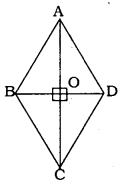Solution:
Data: ABCD is a rhombus.
Here, AB = BC = CD = DA.
Diagonals AC and BD intersects at ’O’.
To Prove: AB2 + BC2 + CD2 + DA2 = AC2 + BD2.
In rhombus diagonals bisects perpendicularly.
∴ ∠AOB = ∠AOD = 90°.
In ⊥∆AOB,
AB2 = AO2 + BO2 ……..(i)
In ⊥∆BOC,
AC2 = BO2 + CO2 ……..(ii)
In ⊥∆COD,
CD2 = OC2 + OD2 ……….(iii)
In ⊥∆AOD,
By Adding equations (i) + (ii) + (iii) + (iv)
AB2 + BC2 + CD2 + DA2 =
= AO2 + BO2 + BO2 + CO2 + CO2 + DO2 + AO2 + OD2
= 2AO2 + 2BO2 + 2CO2 + 2DO2
= 2AO2 + 2CO2 + 2BO2 + 2DO2
Now, RHS = 2AO2 + 2CO2 + 2BO2 + 2DO2
$$=2 \times\left(\frac{1}{2} \mathrm{AC}\right)^{2}+2 \times\left(\frac{1}{2} \mathrm{AC}\right)^{2}+2 \times\left(\frac{1}{2} \mathrm{BD}\right)^{2}+2 \times\left(\frac{1}{2} \mathrm{BD}\right)^{2}$$
$$=2 \times \frac{1}{4} \mathrm{AC}^{2}+2 \times \frac{1}{4} \mathrm{AC}^{2}+2 \times \frac{1}{4} \mathrm{BD}^{2}+2 \times \frac{1}{4} \mathrm{BD}^{2}$$
$$=\frac{1}{2} A C^{2}+\frac{1}{2} A C^{2}+\frac{1}{2} B D^{2}+\frac{1}{2} B D^{2}$$
RHS = AC2 + BD2
∴ LHS = RHS
∴ AB2 + BC2 + CD2 + DA2 = AC2 + BD2.

2.5 Exercise Class 10 Question 8.
In the following figure, O is a point in the interior of a triangle ABC, OD ⊥ BC, OE ⊥ AC, OF ⊥ AB. Show that
i) OA2 + OB2 + OC2 – OD2 – OE2 – OF2 = AF2 + BD2 + CE2
ii) AF2 + BD2 + CE2 = AE2 + CD2 + BF2.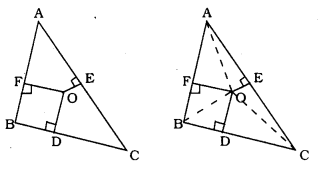Solution:
Data: O is a point in the interior of a triangle ABC, OD ⊥ BC, OE ⊥ AC, OF⊥ AB.
To Proved: i) OA2 + OB2 + OC2 – OD2 – OE2 – OF2 = AF2 + BD2 + CE2
ii) AF2 + BD2 + CE2 = AE2 + CD2 + BF2

(i) OA2 + OB2 + OC2 – OD2 – OE2 – OF2 = AF2 + BD2 + CE2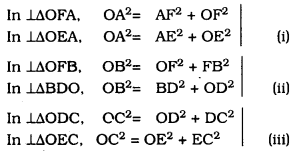OA2 = AF2 + OF2 → (i)
OB2 = BD2 + OD2 → (ii)
OC2 = OE2 + EC2 → (iii)
By Adding equations (i) + (ii) + (iii),
OA2 + OB2+ OC2 = AF2 + OF2 + BD2 + OD2 + OE2 +EC2
∴ OA2 + OB2 + OC2 – OE2 – OF2 – OD2 = AF2 + BD2 + CE2

(ii) AF2 + BD2 + CE2 = AE2 + CD2 + BF2.
OA2 = AF2 + OF2
∴ AF2 = OA2 – OF2 → (i)
OB2 = BD2 + OD2
∴BD2 = OB2 – OD2 → (ii)
OC2 = OE2 + EC2
∴ CE2 = OC2 – OE2 → (iii)
From adding equations (i) + (ii) + (iii),
AF2 + BD2 + CE2 = OA2 – OF2 + OB2 – OD2 + OC2 – OE2
AF2 + BD2 + CE2 = OA2 – OE2 + OB2 – OF2 + OC2 – OE2
∴ AF2 + BD2 + CE2 = AE2 + FB2 + CD2 .

Exercise 2.5 Class 10 Question 9.
A ladder 10 m long reaches a window 8 m above the ground. Find the distance of the foot of the ladder from base of the wall.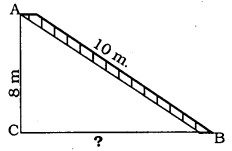Solution:
In ⊥∆ACB, ∠C = 90°, BC = ?
AC2 + CB2 = AB2
(8)2 + CB2 = (10)2
64 + CB2 = 100
CB2 = 100 – 64
CB2 = 36
∴ CB = 6
∴ Ladder is at a distance of 6m from the base of the wall.

2.5 Maths Class 10 Question 10.
A guy wire attached to a vertical pole of height 18 m is 24 m long and has a stake attached to the other end. How far from the base of the pole should the stake be driven so that the wire will be taut?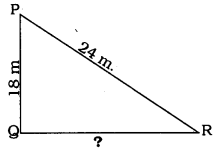Solution:
In ⊥∆PQR, ∠Q = 90°, QR = ?
∴ PQ2 + QR2 = PR2
(18)2 + QR2 = (24)2
324 + QR2 = 576
QR2 = 576 – 324
QR2 = 252
∴ QR = 15.8 m.

Triangles Class 10 KSEEB Solutions Question 11.
An aeroplane leaves an airport and flies due north at a speed of 1000 km per hour. At the same time, another aeroplane leaves the same airport and flies due west at a speed of 1200 km per hour. How far apart will be the two planes after $$1 \frac{1}{2}$$ hours?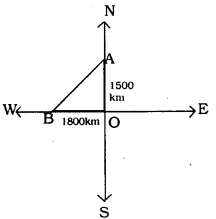Solution:
Distance travelled by aeroplace trowards North is $$1 \frac{1}{2}$$ hours :
$$=1000 \times 1 \frac{1}{2}$$
$$=1000 \times \frac{3}{2}$$
= 1500 km.
Diatance travelled by aeroplane towards West in $$1 \frac{1}{2}$$ Hours :
$$=1200 \times 1 \frac{1}{2}$$
$$=1200 \times \frac{3}{2}$$
= 1800 km.
In ⊥∆AOB,
AB2 = OA2 + OB2
= (1500)2 + (1800)2
= 2250000 + 3240000 = 5490000
$$\mathrm{AB}=\sqrt{5490000}$$
$$A B=\sqrt{90000 \times 61}$$
$$A B=300 \sqrt{61} \mathrm{km}$$ km
∴ Two planes are 300V6T km. apart after 14 hours

10th Maths Triangles Exercise 2.5 Solutions Question 12.
Two poles of heights 6 m and 11m stand on a plane ground. If the distance between the feet of the poles is 12 m, find the distance between their tops.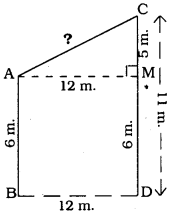Solution:
Pole AB = 6 m.
Pole CD = 11 m.
Distance between poles BD = 12 m.
Distance between feet of the poles, AC = ?
ABDM is a rectangle, AB = MD = 6 m.
BD = AM = 12 m.
In ⊥∆AMC, ∠AMC = 90°
AM = 12 m, CM = 5 m. AC = ?
AC2 = AM2 + CM2
= (12)2 + (5)2
= 144 + 25
AC2 = 169
∴ AC = 13 m.

Triangle Lesson Exercise 2.5 Question 13.
D and E are points on the sides CA and CB respectively of a triangle ABC right angled at C.
Prove that AE2 + BD2 = AB2 + DE2.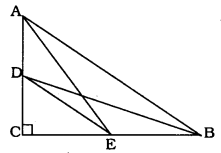Solution:
Data: In ∆ABC, ∠C = 90°, D and E are points on the sides CA and CB respectively
Top Prove: AE2 + BD2 = AB2 + DE2
In ⊥∆ACE, ∠C = 90°
∴ AE2 = AC2 + CE2 ………. (i)
In ⊥∆DEB, ∠C = 90°
∴ BD2 = DC2 + CB2 ………… (ii)
From adding equations (i) + (ii)
AE2 + BD2 = AC2 + CE2 + DC2 + CB2
= AC2 + CB2 + DC2 + CE2
∴ AE2 + BD2 = AB2 + DE2 ( . Theorem 8).

Triangles Class 10 Solutions KSEEB Question 14.
The perpendicular from A on side BC of a ∆ABC intersects BC at D such that DB = 3CD (see the following figure) Prove that 2AB2 = 2AC2 + BC2.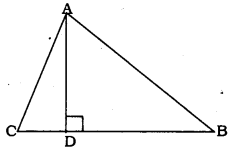Solution:
In ∆ACD,
AD2 = AC2 – DC2……….. (1)
In ∆ABD,
AD2 = AB2 – DB2………. (2)
From equations (1) and (2),
AC2 – DC2 = AB2 – DB2 ………… (3)
3DC = DB (data given)
$$\mathrm{DC}=\frac{\mathrm{BC}}{4}, \text { and } \mathrm{DB}=\frac{3 \mathrm{BC}}{4}$$ ……….. (3)
Substituting eqn. (4) in eqn. (3),
$$\mathrm{AC}^{2}-\left(\frac{\mathrm{BC}}{4}\right)^{2}=\mathrm{AB}^{2}-\left(\frac{3 \mathrm{BC}}{4}\right)^{2}$$
$$A C^{2}-\frac{B C^{2}}{16}=A B^{2}-\frac{9 B C^{2}}{16}$$
$$\frac{16 \mathrm{AC}^{2}-\mathrm{BC}^{2}}{16}=\frac{16 \mathrm{AB}^{2}-9 \mathrm{BC}^{2}}{16}$$
16AC2 – BC2 = 16 AB2 – 9BC2
16AB2 – 16AC2 = 9 BC2 – BC2
16AB2 – 16AC2 = 8BC2
8(2AB2 – 2AC2 = BC2)
2AB2 – 2AC2 = BC2
2AB2 = 2AC2 ≠ BC2

Maths Exercise 2.5 Class 10 Question 15.
In an equilateral triangle ABC, D is a point on side BC such that $$B D=\frac{1}{3} B C$$, Prove that 9AD2 = 7AB2.
Solution:
∆ABC is an equilateral triangle.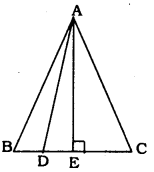AB = BC = AC = a
In ∆ABC, AE is perpendicular line.
$$B E=E C=\frac{B C}{2}$$
Altitude, $$\mathrm{AE}=\frac{\mathrm{a} \sqrt{3}}{2}$$
$$\mathrm{BD}=\frac{1}{3} \mathrm{BC}(\mathrm{Data})$$
$$B D=\frac{a}{3}$$
$$=\quad \frac{a}{2}-\frac{a}{3}=\frac{a}{6}$$
$$=\left(\frac{\mathrm{a} \sqrt{3}}{2}\right)^{2}+\left(\frac{\mathrm{a}}{6}\right)^{6}$$
$$=\frac{3 a^{2}}{4}+\frac{a^{2}}{36}=\frac{27 a^{2}+a^{2}}{36}$$
$$=\frac{28 \mathrm{a}^{2}}{36}$$
$$=\frac{7}{9} \mathrm{a}^{2}$$
$$\mathrm{AD}^{2}=\frac{7}{9} \mathrm{AB}^{2} \quad 9 \mathrm{AD}^{2}=7 \mathrm{AB}^{2}$$

10th Maths Exercise 2.5 Question 16.
In an equilateral triangle, prove that three times the square of one side is equal to four times the square of one of its altitudes.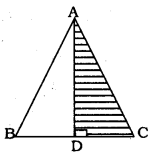Solution:
Data: ABC is an equilateral triangle.
Here, AB = BC = CD.
∴ AC2 = AD2 + DC2
$$=A D^{2}+\left(\frac{1}{2} A C\right)^{2}$$
$$\left( \begin{array}{l}{\mathrm{DC}=\frac{1}{2} \mathrm{BC}} \\ {\mathrm{DC}=\frac{1}{2} \mathrm{AC}}\end{array}\right)$$
$$\mathrm{AC}^{2}=\mathrm{AD}^{2}+\frac{1}{4} \mathrm{AC}^{2}$$
$$\frac{\mathrm{AC}^{2}}{1}-\frac{1}{4} \mathrm{AC}^{2}=\mathrm{AD}^{2}$$
$$\frac{4 \mathrm{AC}^{2}-1 \mathrm{AC}^{2}}{4}=\mathrm{AD}^{2}$$

KSEEB Solutions For Class 10 Maths Question 17.
Tick the correct answer and justify:
In ∆ABC, $$A B=6 \sqrt{3} \mathrm{cm}$$. AC = 12 cm. and BC = 6 cm. The angle B is
A) 120°
b) 60°
C) 90°
D) 45°
Solution:
C) 90°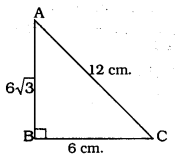Justification:
a             b           c
$$6 \sqrt{3}$$        6             12
Here largest side is 12 cm.
If square of the hypotenuse is equal to square of other two sides, then it is a right angled triangle.
∴ c2 = a2 + b2
AC2 = AB2 + BC2
$$(12)^{2}=(6 \sqrt{3})^{2}+(6)^{2}$$
144 = 36 × 3 + 36
144 = 108 + 36
144 = 144
∴ ∆ABC is a right angled triangle and angle opposite to hypotenuse, ∠B = 90°.

We hope the given KSEEB SSLC Class 10 Maths Solutions Chapter 2 Triangles Ex 2.5 will help you. If you have any query regarding Karnataka SSLC Class 10 Maths Solutions Chapter 2 Triangles Exercise 2.5, drop a comment below and we will get back to you at the earliest.